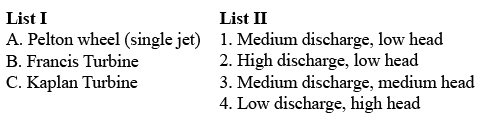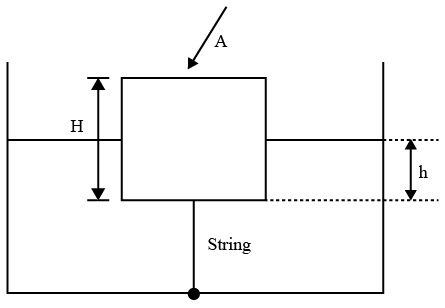# ISRO Scientist or Engineer Mechanical 2011

Instructions

For the following questions answer them individually

Question 1

# Match List I with List If and select the correct answerQuestion 2

# Reversible adiabatic process may be expressed as $$\left(\frac {T_1}{T_2}\right)$$ equal toQuestion 3

# A gas is so expanded in a cylinder that its temperature remains constant. The resulting variation of pressure vs. volume isQuestion 4

# According to first law of thermodynamicsQuestion 5

# Pick up the incorrect statement for centrifugal pumpsQuestion 6

# If the discharge of a centrifugal pump is throttled then its suction liftQuestion 7

# A cylindrical body of cross-sectional area A height H and the density $$\rho_s$$, is immersed to a depth h in a liquid of density $$\rho$$, and tied to the bottom with a string. The tension in the string isQuestion 8

# A manometer méasures the pressure differential between two locations of a pipe carrying water. If the manometric liquid is mercury (specific gravity 13.6) and the manometer showeda level difference of 20 cm, then the pressure head difference of water between the two tapings will beQuestion 9

# Flow takes place at Reynolds Numberof 1500 in two different pipes with relative roughness of 0.001 and 0.002. The friction factorQuestion 10

# A liquid compressedi in cylinder has a volume of $$0.04 m^3$$ at $$50 kg/cm^2 and a volume of$$0.039 m^3$$at$$150 kg/cm^2. The bulk modulus of elasticity of liquid isOR